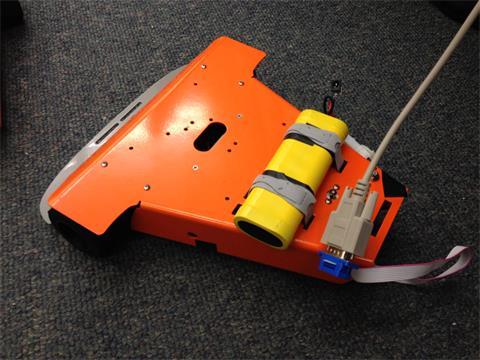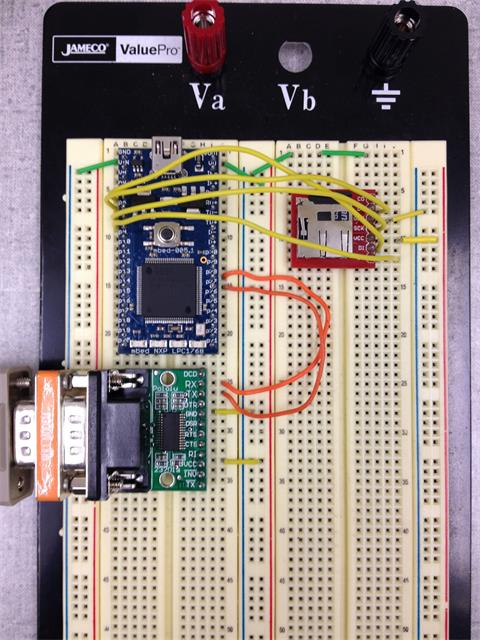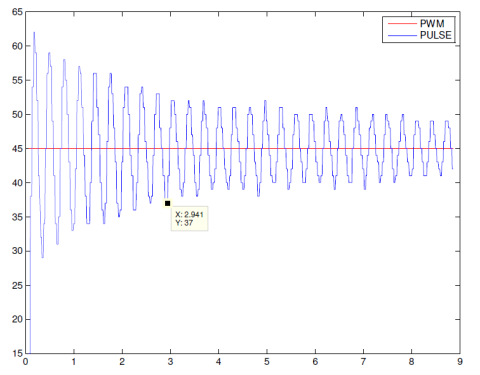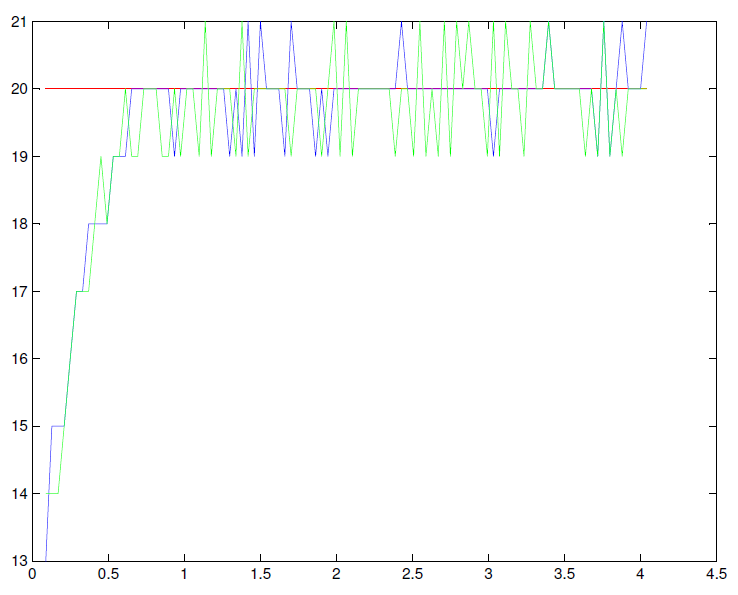## Description

In this project, I controlled the Serializer Stinger using PID control theory. Components for this project are following:

• Serializer Stinger
• Battery for Stinger( 12V - 2A )
• SD card

The purpose of this project is learning and implement PID on a mobile robot base. The mobile robot base will move in a circular repetitive motion. Serializer Stinger is a good robot to start with because it has embedded PID control algorithm. This tutorial assume the views have a basic understanding of programming on mbed and PID control theory.## Wiring Diagram

 MBed Serial Adapter P28 Rx P27 Tx VCC VCC GND GND

Connect serial adapter and Serializer Stinger with serial cable and null modem if necessary.

## Serializer Stinger

Serializer Stinger is a well developed system. The brain is a board having two H-bridge. a micro-controller, Control of the robot is by sending commands through several kinds of protocol. In this project, I use RS232 serial to do this.

Several common commands are introduced here. For all commands, refer to the user manual. The syntax of command is a command name followed by parameters. Every command must be terminated with "Carriage Return" character(<CR>).

1. fw<CR>

check the firmware version.

3. vel<CR>

query the velocity of two wheels.

4. vpid<CR>

Query the velocity PID constants.

5. vpid 10:0:5:10<CR>

Set velocity PID constants

6. mogo 1:30 2:45<CR>

Set the velocity of motor 1 by 30 ticks/seconds and motor 2 by 45 ticks/seconds

7. digo 1:1000:25 2:1000:25<CR>

Move distance 1000 ticks at velocity 25

Equipped with these commands, we're able to control Stinger. Here is a snippet code to send command to Stinger

#### main.cpp

```#include "mbed.h"

Serial uart(p28,p27);

int main() {
while (1) {
wait(1);
uart.baud(19200);//The default baud rate of Stinger is 19200
}
}
```

## Tunning Code Setup

Using mbed to send velocity command to Stinger and read velocity of each motor from Stinger. Using these data plots the system response in Matlab. The partial code for mbed:

#### main.cpp

```    uart.printf("mogo 1:20 2:20\r");//let motor run at velocity 20
t.start();//start the timer
while(1){
uart.printf("vel\r");// Send velocity command
//Get response from Stinger
i = 0;
while( (tmp = uart.getc()) != '>' ){
if( tmp != 13 && tmp != 10 ){
buf[i++] = tmp;
}
}
double jj1 = (buf-'0')*10+(buf-'0');// cast char to int
double jj2 = (buf-'0')*10+(buf-'0');// cast char to int
pc.printf("20 %f %f %f ", jj1,jj2, t.read());// send data to host computer
// The number of data to collect.
if(j < 100){
j++;
}else{
break;
}
}
t.stop();
```

The matlab code to get the data from serial port

#### serial.m

```	s = serial('COM5');
set(s,'InputBufferSize',10240);
set(s,'BaudRate',9600);
if strcmp(s.Status, 'closed')
fopen(s);
end
j = fscanf(s,'%f %f %f %f',[4,2000]);
fclose(s);
delete(s);
clear s;
```

Code to plot data in Matlab

#### plot.m

```	data = transpose(j);
[row col] = size(data);
target = data(:,1);
wheel1 = data(:,2);
wheel2 = data(:,3);
time = data(:,4);
plot(time,target,'r');hold on;
plot(time,wheel1,'b');
plot(time,wheel2,'g');
axis auto;
hold off;
```

## Tuning PID constant

The major job in this project is tuning velocity PID constants. Using feedback loop to control the system is the core of PID control. First of all, let's see what effect each one has on the system:

 Response Rise Time Overshoot Settling Time Kp Decrease Increase None Ki Decrease Increase Increase Kd None Decrease Decrease

Using this table as a guide.

There're several common methods to tuning. The one I used here is called Ziegler-Nichols. Comparing with other tuning method, this method is simple to do for beginners.

The first step is adjust Kp value until the system oscillate. For example:From this plot, we need to measure the oscillation period ( T ).

Then calculate Kp, Ki and Kd according to the table:

 Controller Kp Ki Kd P 0.5*Kp 0 0 PI 0.45*Kp Kp/0.85*T 0 PID 0.6*Kp Kp/(0.5*T) Kp*T*0.12

Since we are implementing PID, we using the third row to get PID constants. Applying these constants to Stinger using "vpid " command.

The new running plot looks a lot better:Normally, the result won't be this nice. These values might need more tuning. If that's the case, the table at the beginning of this section come to serve. Adjusting based on ones' need. There's no universal perfect PID constants.